Question

What is the equivalent recursive definition for an = 12+ (n – 1)3?
A. a1 = 3, An = An-1 + 12
B. a1 = 12, An = 30n-1
C. a1 = 12, Un = On-1 +3
D. a1 = n, an= 1201-1+3

1.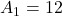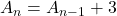Step-by-step explanation:

Given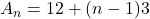Required

Write as recursive

We have:Open bracket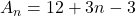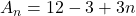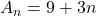Calculate few terms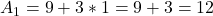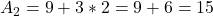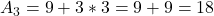The above shows that the rule is to add 3.

So, we have: